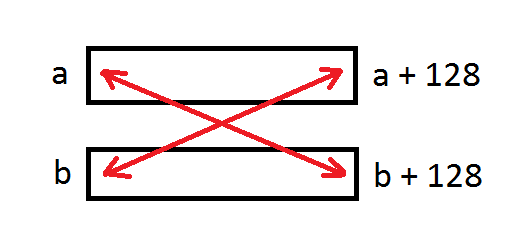# Vectorization part5. Multiversioning by data dependency.

Categories: vectorization

## 03 Nov 2017

Subscribe to my newsletter, support me on Patreon or by PayPal donation.

Vectorization doesn’t always come for free. In this post we will see what penalties we have to pay with vectorization.

Without further ado let me show you example of the code:

``````void foo( unsigned short * a, unsigned short * b )
{
for( int i = 0; i < 128; i++ )
{
a[i] += b[i];
}
}
``````

Now lets consider a kind of weird invocation of this function:

``````  unsigned short x[] = {1, 1, 1, 1, ... , 1}; // 129 elements
unsigned short* a = x + 1;
unsgined short* b = x;
foo (a, b);
``````

In scalar version we will receive results: `x = {1, 2, 3, 4, 5, ... }`. But in vector version we will first load some portion of a (starting from x + 1). Then we will load some portion of b (starting from x). Then we will add two vector registers together, resulting in `x = (2, 2, 2, 2, ...)`. Oops! Something is wrong.

Vectorized version of the loop works perfectly fine as long as input arrays do not alias (there is memory intersection).

To protect from this problem compilers insert runtime checks for arrays aliasing. Lets see what clang 5.0 generated for us (link to godbolt):

``````  lea rax, [rsi + 256] # calculating the end of b (b + 128)
cmp rax, rdi         # comparing the beginning of a and the end of b
jbe .LBB0_4
lea rax, [rdi + 256] # calculating the end of a (a + 128)
cmp rax, rsi         # comparing the beginning of b and the end of a
jbe .LBB0_4
xor eax, eax
<scalar version>
.LBB0_4:
<vector version>
``````

As you can see there is some runtime dispatching between scalar and vector version of the same loop. This is what is called Multiversioning.Normally pointer aliasing is rather rare case, but we don’t know for sure, so we need to have a runtime check for that. If you are sure that your arrays will never alias you can use `__restrict__` keyword to tell the compiler about it: (link to godbolt). As you can see runtime check was removed.

Situation gets somewhat complex when there are multiple arrays in the function arguments. This significantly increases the number of runtime checks in the beginning of the function. Gcc even has heuristic for that: `--param vect-max-version-for-alias-checks` which is 10 by default.

Another frequently used runtime check for the compiler is testing number of loop iterations. It should not be negative or lower than the vectorization width.

### All posts from this series:

1. Vectorization intro.
2. Vectorization warmup.
3. Checking compiler vectorization report.
4. Vectorization width.